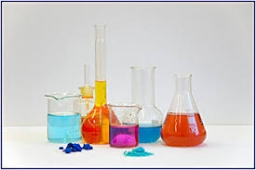# Solution 19283

How many grams of 80% solution and 55% solution do we need to mix to obtain 100 grams of 60% solution?

a =  20
b =  80

### Step-by-step explanation:

0.80a+0.55b=0.60·100
a+b=100

0.80·a+0.55·b=0.60·100
a+b=100

0.8a+0.55b = 60
a+b = 100

Pivot: Row 1 ↔ Row 2
a+b = 100
0.8a+0.55b = 60

Row 2 - 0.8 · Row 1 → Row 2
a+b = 100
-0.25b = -20

b = -20/-0.25 = 80
a = 100-b = 100-80 = 20

a = 20
b = 80

Our linear equations calculator calculates it.Did you find an error or inaccuracy? Feel free to write us. Thank you!

Tips for related online calculators